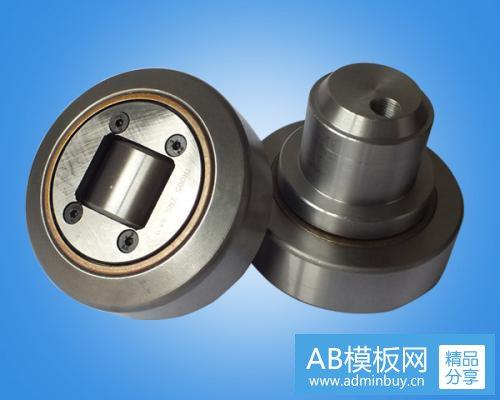Contact Us

# 滚轮滚针轴承的最大Lyapunov指数、奇怪吸引子及关

1.滚轮滚针轴承(bearing)的最大Lyapunov指数Tm =式中，Tm为可预测(predict)时间，不为最大Lyapunov指数。

2.奇怪吸引子

3.关联维数
(z(i+(k -1)r)-z(1+(k -1))2

r(.)=点

InC(r,m)D.(r,m)=lim in,

C(r,m)=-N(N-1) 142

Heaviside函数的定义为
(-r(i,)=1, r>r(,)，r<r(i,I)